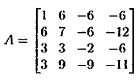Terry Tung>試卷(2022/05/13)

# [無官方正解]主題課程_對角化：特徵值、特徵向量、特徵空間#107848

1.

110台大電信丙組
(10%) Consider a matrixWhich of the following is an eigenvalue of this matrix M?
(A)1
(B)-3
(C)9
(D) -1+2j
(E) -1+4j

2.

(10%) Consider the matrix M in. It is known that M has four distinct eigernvalues, and let us denote the four cotresponding eigenvectors asWhich of the following matrices also hasas its eigenvectors?
(A)(B)(C)(D)(E)3.109台大電信乙組、資料科學_工數D
(5%) Which of the following is an eigenvector of A =?
(A) x=(B) x=(C)x=(D) x=；
(E) None of the above.

4.109中山_電機工數乙
Let A. Which of the following statements are true?
(A) If A is singular, then O is an eigenvaluc of A.
(B) A and AT share the same eigenvalues and eigenvectors.
(C) If A is diagonalizable, then A has n distinct eigenvalues.
(D) Suppose that A is nonsingular. The condition Ax = λλx implies(E) Suppose that all the eigenvalues of A are real and positive. Then we have det( A ) ＞ 0.

5.108台大電機資訊學院_工數C
.(10%) For the matrix below, which of the following are correct?(A)-1 is its eigenvalue.
(B) 1 is its eigenvalue.
(C)-3 is its eigenvalue.
(D)Zero vector belongs to its eigenvector.
(E)[1 0 2]T is a basis of its eigenspace.

6.108台大電機資訊學院_工數C
(5%) Which of the following are correct?
(A) An eigenvalue of a matrix has infinite number of eigenvectors.
(B) If det(A -λln) = O, λ is the eigenvalue of n x n matrix A.
(C) IfA is the cigenvalue of matrix A, the columns of (A - λIn) are independent.
(D) Ifa is the eigenvalue of matrix A, there exist a non-zero vector V such that Av = av.
(E) The number of the eigenvalue of n x n matrix A may larger than n.

【非選題】
7.
109台大電信丙組
9.(18%) Letwhose eigenvalues are -5, -2 and l.

【題組】 (a) (6%) Plcase find the eigenvalues of.

【非選題】
8.【題組】(b) (6%) Please find the eigenvalues of 2A.

【非選題】
9.【題組】(c) (6%) Please find the eigenvalues of A2

【非選題】
10.109中興電機 (15 pts)
The eigenvalues of A and AT are the same, because det(A- λ l) -det(A-  λ l)T= det(AT-  λ l). By coming up with a 2 X 2 counter-example, show an example that the cigenvectors of A and ATneed not be the same.

【非選題】
11.106中興電機
(10pts) Suppose A is a real and symmetric matrix with order n, and has a repeated eigenvalue. Then for every i in {1,2, ... n}, there exists an eigenvector whose ith component is 0.

【非選題】
12.105中興電機
(10pts) Suppose A and B are two matrices with size m✕n and n✕m resbectively. Show that the nonzero eigenvalues of AB and BA are the same.